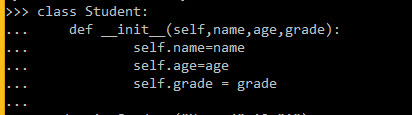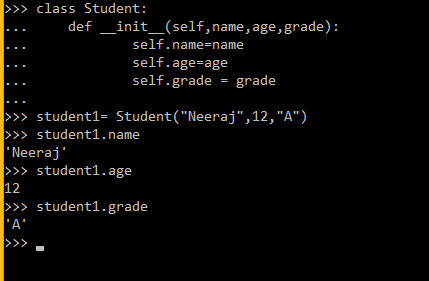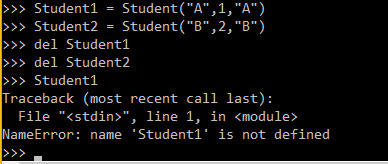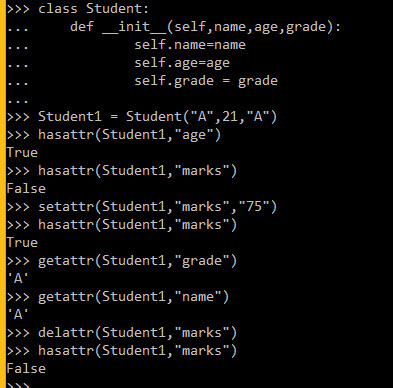# Python Class

## Introduction

In this chapter, we will learn to use Python Class.

## Class

Create Class:

1. Firstly open python terminal and in Command Prompt of Windows and write code as below:

class Student:

• self.name=name
• self.age=ageIn the above image __init__ is default function of python. It also works as a constructor of the parameter in python. We can give many parameters to this function. Self parameter work as an object to show all methods and variables.

2. Now make Object of class and insert a value of Student like below:

Student1 = Student(“Neeraj”,12,”A”)Class Attributes:

These Class attributes are built-in attributes that can be used for a specific task.

• __dict__: Dictionary containing the class's namespace.
• __doc__: Class documentation string or none, if undefined.
• __name__: Class name.
• __module__: Module name in which the class is defined. This attribute is "__main__" in interactive mode.
• __dict__: A possibly empty tuple containing the base classes, in the order of their occurrence in the base class list.

3. Now we example of all the above attributes.
• print(Student.__doc__)
• print(Student.__name__)
• print(Student.__module__)
• print(Student.__bases__)
• print(Student.__dict__)These define the Class Concept according to their functionality.

4. Creation and Deletion of Object is easy in python as below code:
• Student1 = Student("A",1,"A") // create student1 object
• Student2 = Student("B",2,"B") // “”
• del Student1 // delete the object student1
• del Student2 //””Functions in Classes:

class Student:

a. self.name=name
b. self.age=age

• def displayStudent(self):
a. return ("Student name is "+self.name+" and age is "+str(self.age))

• student1=Student("BOB",21,"A")
• student1.displayStudent()

Output: 'Student name is BOB and age is 21'

Class Method Built-In:

• getattr(obj, name[, default]) : to access the attribute of object.
• hasattr(obj,name) : to check if an attribute exists or not.
• setattr(obj,name,value) : to set an attribute. If the attribute does not exist, then it would be created.
• delattr(obj, name) : to delete an attribute

5. Let's take an example of these Methods

class Student:

• def __init__(self,name,age

• self.name=name
• self.age=age
• Student1 = Student("A",21,"A")
• hasattr(Student1,"age")
• hasattr(Student1,"marks")
• setattr(Student1,"marks","75")
• hasattr(Student1,"marks")